# Schrödinger equation

(diff) ← Older revision | Latest revision (diff) | Newer revision → (diff)

A fundamental equation in quantum mechanics that determines, together with corresponding additional conditions, a wave function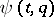characterizing the state of a quantum system. For a non-relativistic system of spin-less particles it was formulated by E. Schrödinger in 1926. It has the form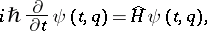where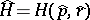is the Hamilton operator constructed by the following general rule: in the classical Hamilton function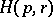the particle momentaand their coordinatesare replaced by operators that have, respectively, the following form in the coordinate representation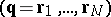and in the momentum representation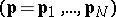: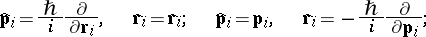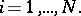For charged particles in an electromagnetic field, characterized by a vector potential, the quantity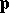is replaced by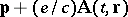. In these representations the Schrödinger equation is a partial differential equation, for example, for particles in the potential field,Discrete representations are possible, in which the functionis a multi-component function and the operator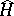has the form of a matrix. If a wave function is defined in the space of occupation numbers, then the operatoris represented by some combinations of creation and annihilation operators (the second quantization representation, cf. Annihilation operators; Creation operators).

The generalization of the Schrödinger equation to the case of a non-relativistic particle with spin(a two-component function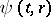) is called the Pauli equation (1927); to the case of a relativistic particle with spin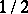(a four-component function) — the Dirac equation (1928); to the case of a relativistic particle without spin — the Klein–Gordon equation (1926); with spin 1 (the functionis a vector) — the Proca equation (1936); etc.

The solution of the Schrödinger equation is defined in the class of functions that satisfy the normalization condition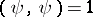for all(the brackets mean integration or summation over all values of). To find the solution it is necessary to formulate initial and boundary conditions, corresponding to the character of the problem under consideration. The most characteristic among such problems are:

1) The stationary Schrödinger equation and the determination of admissible values of the energy of the system. Assuming that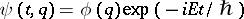and requiring in conformity with the normalization condition and the condition of absence of flows at infinity that the wave function and its gradients vanish when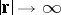, one obtains an equation for the eigenvalues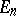and eigenfunctions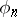of the Hamilton operator: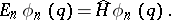Characteristic examples of the exact solution to this problem are: the eigenfunctions and energy levels for a harmonic oscillator, a hydrogen atom, etc.

2) The quantum-mechanical scattering problem. The Schrödinger equation is solved under boundary conditions that correspond at a large distance from the scattering centre (described by the potential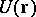) to the plane waves falling on it and the spherical waves arising from it. Taking into consideration this boundary condition, the Schrödinger equation can be written as an integral equation, the first iteration of which with respect to the term containingcorresponds to the so-called Born approximation. This equation is also called the Lippman–Schwinger equation.

3) The case where the Hamiltonian of the system depends on time,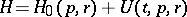, is usually considered in the framework of time-dependent perturbation theory. This is a theory of quantum transition, the determination of the system's reaction to an external perturbation (dynamic susceptibility) and characteristics of relaxation processes.

To solve the Schrödinger equation one usually applies approximate methods, regular methods (different types of perturbation theories), variational methods, etc.

How to Cite This Entry:
Schrödinger equation. Encyclopedia of Mathematics. URL: http://encyclopediaofmath.org/index.php?title=Schr%C3%B6dinger_equation&oldid=14193
This article was adapted from an original article by I.A. Kvasnikov (originator), which appeared in Encyclopedia of Mathematics - ISBN 1402006098. See original article